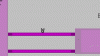# GMLGame Jitters?

#### Abox

##### Member
Heya! I've been working on my project and I've had this problem for a bit.
I've tried everything I could think of but none of them worked. My problem is when the player jumps, the jump looks really Jittery. The parallax background is also Jittery when the player moves, when the player stops in snaps to the player.
If anyone knows the fix to this please let me know!!Code:
``````///React to inputs
scr_get_input();
move = key_left + key_right;

//Move Right
if (move == 1)
{
hspd += acc;
if (hspd >= maxspeed) hspd = maxspeed;
} else if (hspd > 0)
{
hspd -= acc;
if (hspd <= 0) hspd = 0;
}
//Move Left
if (move == -1)
{
hspd -= acc;
if (hspd <= -maxspeed) hspd = -maxspeed;
} else if (hspd < 0)
{
hspd += acc;
if (hspd >= 0) hspd = 0;
}

//Running
if key_run
{
maxspeed = 3;
} else if !key_run
{
maxspeed -= 0.1;
if maxspeed < 2 maxspeed = 2;
}

//Gravity
if (vspd < 10) vspd += grav;

if (place_meeting(x,y+1,obj_solid))
{
if (key_jump)
{
vspd = -jumpspeed
audio_play_sound(snd_jump,5,false)
}
}

if (vspd < 0) && (!key_jump_held)
{
vspd = max(vspd,-jumpspeed/3)

}``````
Background Parallax
Code:
``````//Horizontal Parallax
background_x = floor(view_xview/1.2);
background_x = floor(view_xview/1.4);
background_x = floor(view_xview/1.6);
background_x = floor(view_xview/1.8);

//Verticle Parallax
background_y = floor(view_yview/1.2);
background_y = floor(view_yview/1.4);
background_y = floor(view_yview/1.6);
background_y = floor(view_yview/1.8);``````

#### Attachments

• 122.6 KB Views: 0

#### TsukaYuriko

##### Q&A Spawn Camper
Forum Staff
Moderator
How are you drawing the player? Make sure you round or floor the coordinates before drawing, as drawing at sub-pixels may introduce blurring or distortion.

#### Abox

##### Member
How are you drawing the player? Make sure you round or floor the coordinates before drawing, as drawing at sub-pixels may introduce blurring or distortion.
I don't have a draw event tied to the player, should I do one and do draw_self(); ?

#### robproctor83

##### Member
You would replace draw self with draw sprite and round out the x and y or round the x and y vars and use draw self.

#### Abox

##### Member
You would replace draw self with draw sprite and round out the x and y or round the x and y vars and use draw self.
So something like this? `draw_sprite(draw_self(),0,floor(x),floor(y))`

#### Nidoking

##### Member
No, draw_self is a function that draws the instance, the same way that draw_sprite is a function that draws a sprite. You need to tell it which sprite to draw. Presumably, you can get that with sprite_index.

•Abox

#### Abox

##### Member
Looks like it works fine now! No more jitter for the player, thank you so much!!
All I need to do now is figure out the background bit

#### robproctor83

##### Member
It's probably the same issue, just on the BG.